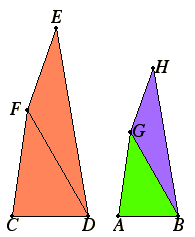# Proposition 18

To describe a rectilinear figure similar and similarly situated to a given rectilinear figure on a given straight line.

Let AB be the given straight line and CE the given rectilinear figure.

It is required to describe on the straight line AB a rectilinear figure similar and similarly situated to the rectilinear figure CE.I.23

Join DF. Construct the angle GAB equal to the angle at C, and the angle ABG equal to the angle CDF, on the straight line AB at the points A and B on it.

I.32

Therefore the remaining angle CFD equals the angle AGB. Therefore the triangle FCD is equiangular with the triangle GAB.

Therefore, proportionally, FD is to GB as FC is to GA, and as CD is to AB.

I.23

Again, construct the angle BGH equal to the angle DFE, and the angle GBH equal to the angle FDE, on the straight line BG and at the points B and G on it.

Therefore the remaining angle at E equals the remaining angle at H. Therefore the triangle FDE is equiangular with the triangle GBH. Therefore, proportionally, FD is to GB as FE is to GH, and as ED is to HB.

V.11

But it was also proved that FD is to GB as FC is to GA, and as CD is to AB. Therefore FC is to AG as CD is to AB, and as FE is to GH, and further as ED is to HB.

And, since the angle CFD equals the angle AGB, and the angle DFE equals the angle BGH, therefore the whole angle CFE equals the whole angle AGH.

For the same reason the angle CDE also equals the angle ABH.

And the angle at C also equals the angle at A, and the angle at E equals the angle at H.

VI.Def.1

Therefore AH is equiangular with CE, and they have the sides about their equal angles proportional. Therefore the rectilinear figure AH is similar to the rectilinear figure CE.

Therefore the rectilinear figure AH has been described similar and similarly situated to the given rectilinear figure CE on the given straight line AB.

Q.E.F.

## Guide

It is evident from the diagram, if not from the text, that AB should correspond to CD.

Although the figure has only four sides, it is clear that the method applies to figures with more than four sides.

This proposition is used in the proofs of propositions VI.22, VI.25, and VI.28, and the corollary is used in XII.17.